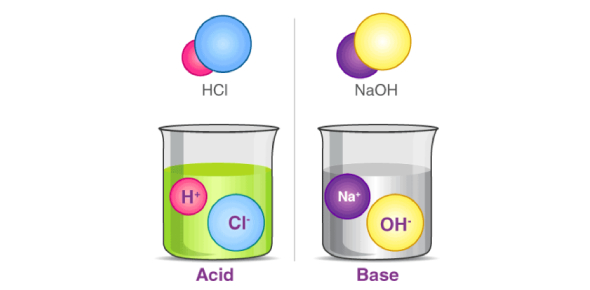# Test Your Acid-base Knowledge Quiz

20 Questions | Total Attempts: 1344SettingsCreate your own QuizIn chemistry class over the past days we have covered acids and bases, how to write chemical equations and what we get when we mixes the two. The quiz below is designed to test your Acid-base knowledge and ensure you are ready for any question posed to you on the topic. Give it a try and see what you score.

• 1.
An acid will dissociate (release) _______ ions in water.
• A.

Hydrogen

• B.

Hydroxide

• C.

Chloride

• D.

Oxide

• 2.
A base will dissociate (release) __________ ions in water.
• A.

Hydrogen

• B.

Hydroxide

• C.

Chloride

• D.

Oxide

• 3.
An acid's pH is
• A.

Less than 7

• B.

Equal to 7

• C.

Greater than 7

• 4.
A base's pH is
• A.

Less than 7

• B.

Equal to 7

• C.

Greater than 7

• 5.
A neutral solution has a pH ________
• A.

Less than 7

• B.

Equal to 7

• C.

Greater than 7

• 6.
Bases taste
• A.

Sweet

• B.

Sour

• C.

Tangy

• D.

Bitter

• 7.
An indicator changes color based on changes in
• A.

Amount of liquid

• B.

Density

• C.

PH

• 8.
PH paper is a good indicator because
• A.

It has a vial to match pH values

• B.

It changes a wide variety of colors to indicate the pH

• C.

It is easy to use

• D.

It is paper not a liquid

• 9.
Poinsettia pigment is better indicator than pH paper.
• A.

True

• B.

False

• 10.
Acids can cause burns, bases cannot.
• A.

True

• B.

False

• 11.
A volume of 543mL of 0.5M HCl neutralizes a solution of 0.75M NaOH. What volume of NaOH was neutralized? Ma * Va = Mb * Vb
• A.

203.625mL

• B.

362mL

• C.

814.5mL

• D.

135.75mL

• 12.
A volume of 55mL of 2.0M NaOH is neutralized with 230mL of HCl. What is the concentration of the HCl? Ma * Va = Mb * Vb
• A.

0.48M

• B.

25300M

• C.

8.36M

• D.

87.5 M

• 13.
Which of the following is a BASE?
• A.

HCl

• B.

HF

• C.

KOH

• D.

HBr

• 14.
Neutralization is when
• A.

There is more acid than base

• B.

There is more base than acid

• C.

There is equal amounts of acid and base

• D.

Has nothing to do with acid/base

• 15.
If you are performing a neutralization reaction and you forget to add an indicator (such as phenolthalein) what will happen?
• A.

Your solution will turn too pink

• B.

It doesn't matter, you don't need an indicator

• C.

You will not be able to tell when it is neutralized because there is no color change

• D.

It will only turn color if it is really acidic or really basic

• 16.
If you are performing a neutralization reaction, using phenolphthalein, and you add too much base your solution will
• A.

Be clear

• B.

Smell

• C.

Be too pink

• D.

Be dark blue

• 17.
In the equation Ma * Va = Mb * Vb        Ma stands for
• A.

Mass of acid

• B.

Density of acid

• C.

Concentration of acid

• D.

Volume of acid

• 18.
Bases turn ___________ in litmus.
• A.

Clear

• B.

Pink

• C.

Blue

• D.

Green

• 19.
Acids taste
• A.

Sweet

• B.

Sour

• C.

Bitter

• D.

Tangy

• 20.
A volume of 87mL of 1.0M NaOH is neutralized with 65mL of HCl. What is the concentration of the HCl? Ma * Va = Mb * Vb
• A.

1.3M

• B.

0.75M

• C.

22M

• D.

152 M

Related TopicsBack to top
×

Wait!
Here's an interesting quiz for you.